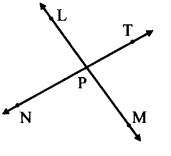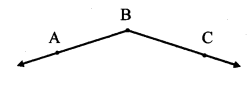# Maharashtra Board Class 7 Maths Solutions Chapter 4 Angles and Pairs of Angles Practice Set 18

## Maharashtra State Board Class 7 Maths Solutions Chapter 4 Angles and Pairs of Angles Practice Set 18

Question 1.
Name the pairs of opposite rays in the figure alongside.Solution:

1. Ray PL and ray PM
2. Ray PN and ray PT

Question 2.
Are the ray PM and PT opposite rays? Give reasons for your answer.Solution:
No.
Ray PM and Ray PT do not form a straight line and hence are not opposite rays.

Maharashtra Board Class 7 Maths Chapter 4 Angles and Pairs of Angles Practice Set 18 Intext Questions and Activities

Question 1.
Observe the adjacent figure and answer the following questions. (Textbook pg. no. 28)1. Name the rays in the figure alongside.
2. Name the origin of the rays
3. Name the angle in the given figure

Solution:

1. Ray BA and ray BC
2. Point B
3. ∠ABC or ∠CBA

Question 2.
Observe the adjacent figure and answer the following questions. (Textbook pg. no. 28)1. Name the angle in the figure alongside.
2. Name the rays whose origin is point B

Solution:

1. ∠ABC or ∠CBA
2. Ray BA and ray BC